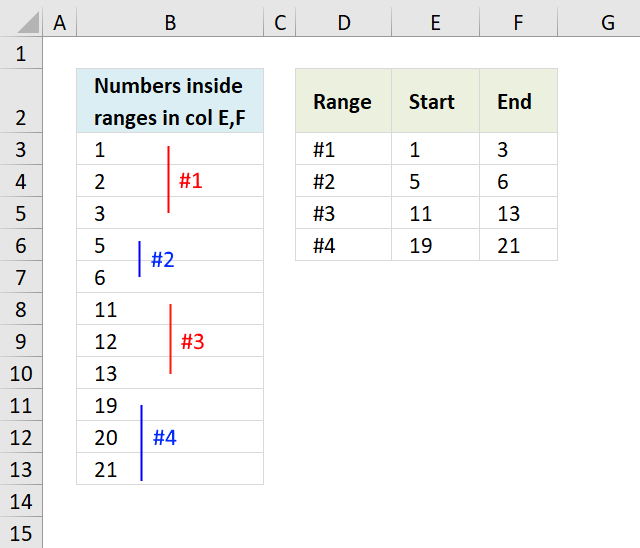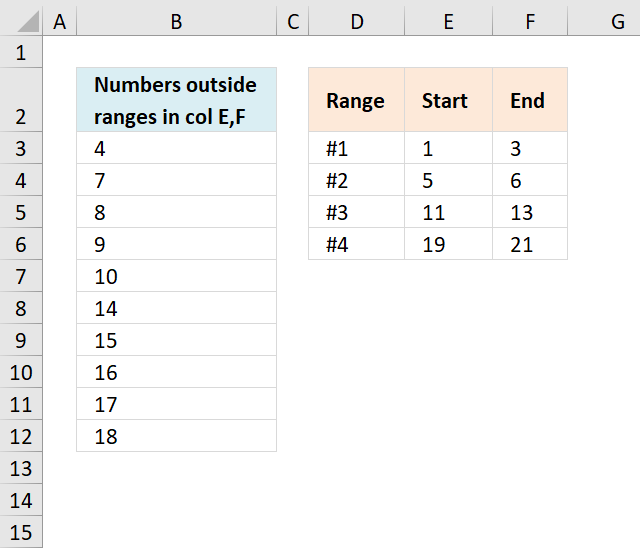Author: Oscar Cronquist Article last updated on December 28, 2018The image above shows an array formula in cell B3 that calculates numbers based on the numerical ranges in cell range E3:F6.

Array formula in B3:

=SMALL(IF(COUNTIFS(\$E\$3:\$E\$6, "<="&ROW(\$1:\$21),\$F\$3:\$F\$6, ">="&ROW(\$1:\$21)), ROW(\$1:\$21)), ROW(A1))

To enter an array formula, type the formula in a cell then press and hold CTRL + SHIFT simultaneously, now press Enter once. Release all keys.

The formula bar now shows the formula with a beginning and ending curly bracket telling you that you entered the formula successfully. Don't enter the curly brackets yourself.

### Explaining formula in cell B3

#### Step 1 - Create a sequence

The ROW function returns a row number based on a cell reference, if the cell reference has multiple rows then the row function returns an array of numbers.

ROW(\$1:\$21)

returns

{1; 2; 3; 4; 5; 6; 7; 8; 9; 10; 11; 12; 13; 14; 15; 16; 17; 18; 19; 20; 21}

#### Step 2 - Check if number is in sequence

The COUNTIFS function checks if a number is larger or equal to the start value and smaller or equal to the end value. If both conditions are met the COUNTIFS function returns 1.

COUNTIFS(\$E\$3:\$E\$6, "<="&ROW(\$1:\$21),\$F\$3:\$F\$6, ">="&ROW(\$1:\$21))

becomes

COUNTIFS(\$E\$3:\$E\$6, "<="&{1; 2; 3; 4; 5; 6; 7; 8; 9; 10; 11; 12; 13; 14; 15; 16; 17; 18; 19; 20; 21},\$F\$3:\$F\$6, ">="&{1; 2; 3; 4; 5; 6; 7; 8; 9; 10; 11; 12; 13; 14; 15; 16; 17; 18; 19; 20; 21})

becomes

COUNTIFS({1;5;11;19}, "<="&{1; 2; 3; 4; 5; 6; 7; 8; 9; 10; 11; 12; 13; 14; 15; 16; 17; 18; 19; 20; 21},{3;6;13;21}, ">="&{1; 2; 3; 4; 5; 6; 7; 8; 9; 10; 11; 12; 13; 14; 15; 16; 17; 18; 19; 20; 21})

and returns

{1; 1; 1; 0; 1; 1; 0; 0; 0; 0; 1; 1; 1; 0; 0; 0; 0; 0; 1; 1; 1}

Tip! Use an Excel defined Table to create dynamic cell references that you don't have to adjust if more ranges are added or deleted.

#### Step 3 - Return number if in range

The IF function has three arguments, the first one must be a logical expression. If the expression evaluates to TRUE  then one thing happens (argument 2) and if FALSE another thing happens (argument 3).

IF(COUNTIFS(\$E\$3:\$E\$6, "<="&ROW(\$1:\$21),\$F\$3:\$F\$6, ">="&ROW(\$1:\$21)), ROW(\$1:\$21))

becomes

IF({1; 1; 1; 0; 1; 1; 0; 0; 0; 0; 1; 1; 1; 0; 0; 0; 0; 0; 1; 1; 1}, ROW(\$1:\$21))

becomes

IF({1; 1; 1; 0; 1; 1; 0; 0; 0; 0; 1; 1; 1; 0; 0; 0; 0; 0; 1; 1; 1}, {1; 2; 3; 4; 5; 6; 7; 8; 9; 10; 11; 12; 13; 14; 15; 16; 17; 18; 19; 20; 21})

and returns

{1; 2; 3; FALSE; 5; 6; FALSE; FALSE; FALSE; FALSE; 11; 12; 13; FALSE; FALSE; FALSE; FALSE; FALSE; 19; 20; 21}.

#### Step 4 - Extract k-th smallest number

The ROWS function keeps track of the numbers based on an expanding cell reference. It will expand as the formula is copied to the cells below.

SMALL(IF(COUNTIFS(\$E\$3:\$E\$6, "<="&ROW(\$1:\$21),\$F\$3:\$F\$6, ">="&ROW(\$1:\$21)), ROW(\$1:\$21)), ROWS(\$A\$1:A1))

becomes

SMALL({1; 2; 3; FALSE; 5; 6; FALSE; FALSE; FALSE; FALSE; 11; 12; 13; FALSE; FALSE; FALSE; FALSE; FALSE; 19; 20; 21}, ROWS(\$A\$1:A1))

becomes

SMALL({1; 2; 3; FALSE; 5; 6; FALSE; FALSE; FALSE; FALSE; 11; 12; 13; FALSE; FALSE; FALSE; FALSE; FALSE; 19; 20; 21}, 1)

and returns 1 in cell B3.The array formula in cell B3 lists numbers not in ranges specified in cell range E3:F6.

Array formula in B3:

=SMALL(IF(FREQUENCY(IF((COLUMN(\$B2:\$U2)>\$F\$3:\$F\$6)+ (COLUMN(\$B2:\$U2)<\$E\$3:\$E\$6), COLUMN(\$B2:\$U2), ""), COLUMN(\$B2:\$U2))=ROWS(\$E\$3:\$E\$6), ROW(\$2:\$22), ""),ROW(A1))

### Get the Excel filenumbers-inside-range.xlsx